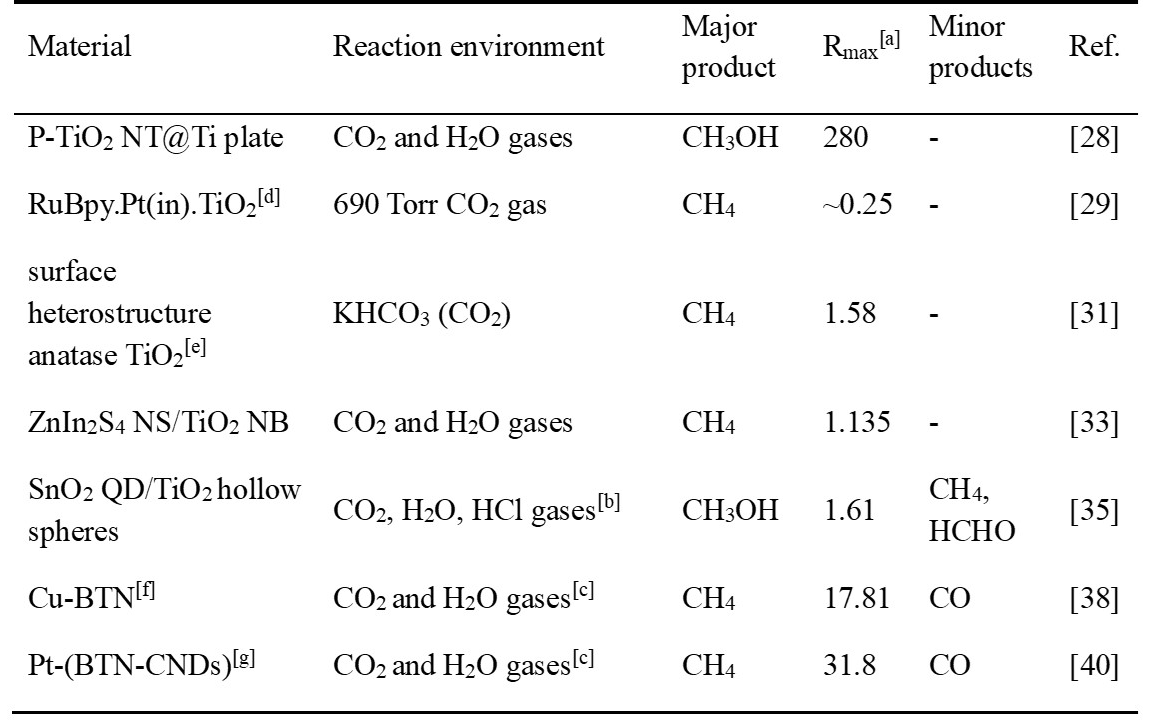0%

# ABSTRACT

Greenhouse gas emission mostly accounts for global warming effect, hence the urgency to realize $\text{CO}_{2}$ removal efficiently and harmlessly to the environment. Base on all of these requirements, utilization of abundant solar energy to achieve $\text{CO}_{2}$ reduction seems the be the best choice. In this review, mechanisms of $\text{CO}_{2}$ photoreduction is briefly introduced, including electrochemical potential analysis and chemical reaction mechanism. Efficiency measurements in this filed are summarized, mainly divided into two groups: catalyst-based measurement and light-based measurement. Applications of $\text{TiO}_{2}$ on $\text{CO}_{2}$ reduction will follow. Subsequently, the modifications on $\text{TiO}_{2}$ specifically for its efficiency and selectivity are discussed in details.

Table 1. Different modifications on $\text{TiO}_{2}$ for $\text{CO}_{2}$ reduction.[a] Maximum formation rate reported for the major product(s), in $\text{μmol} \cdot \text{g}^{-1} \text{h}^{-1}$, unless stated otherwise. [b] Generated by $\text{HCl}$ and $\text{NaHCO}_\text{3}$. [c] Generated by $\text{H}_\text{2}\text{SO}_\text{4}$ and $\text{NaHCO}_\text{3}$. [d] $\text{RuBpy.Pt(in).TiO}_\text{2}$: dye-sensitized $\text{Pt}$ promoted $\text{TiO}_{2}$. RuBpy is a kind of dye molecule, expressed as Tris(2,2’–bipyridyl) ruthenium(II) chloride Molecular. [e] 51% (001) and 49% (101) facets exposing ultrafine anatase $\text{TiO}_{2}$. [f] $\text{Cu}$ decorated brookite $\text{TiO}_{2}$ quasi nanocubes ($\text{BTN}$). [g] $\text{Pt}$ loaded on a substrate made of brookite $\text{TiO}_{2}$ quasi nanocubes ($\text{BTN}$) and $\text{g-C}_\text{3} \text{N}_\text{4}$ nanodots ($\text{CND}$).